## An open box is to be made out of a piece of  cardboard measuring (24 cm × 24 cm) by cutting  of equal squares from the corners and turning up  the sides.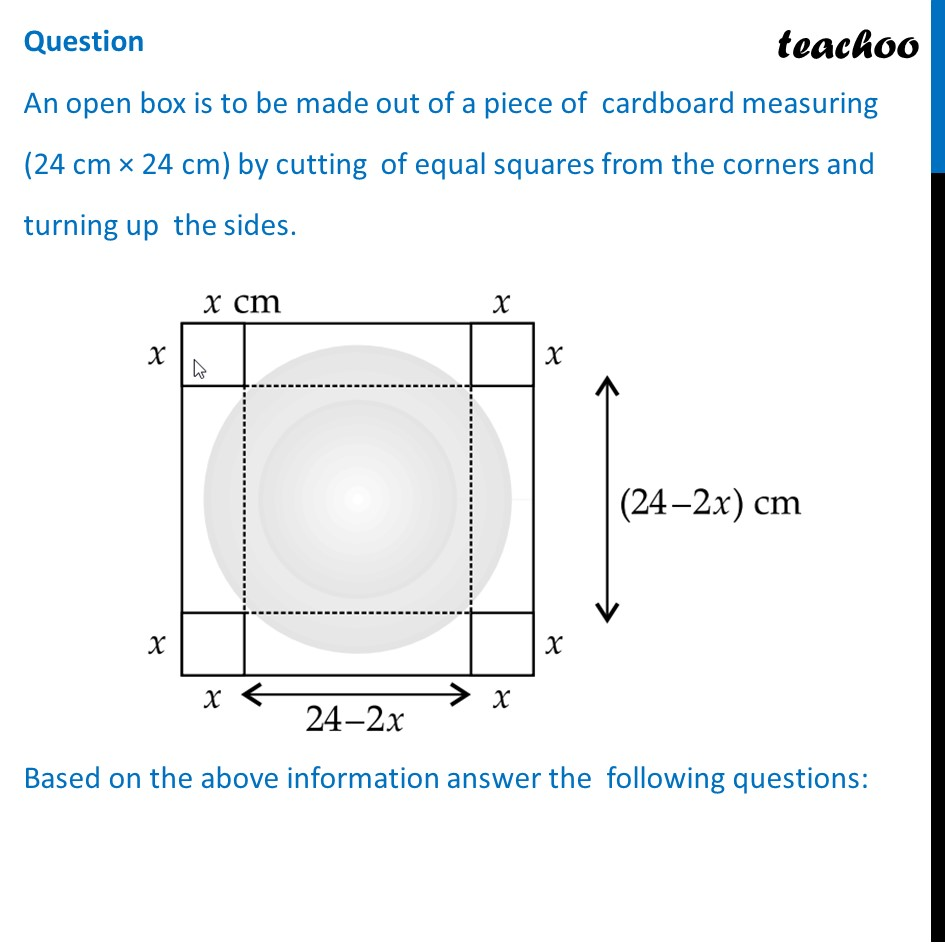## (d) 2x 3 + 48x 2 + 288x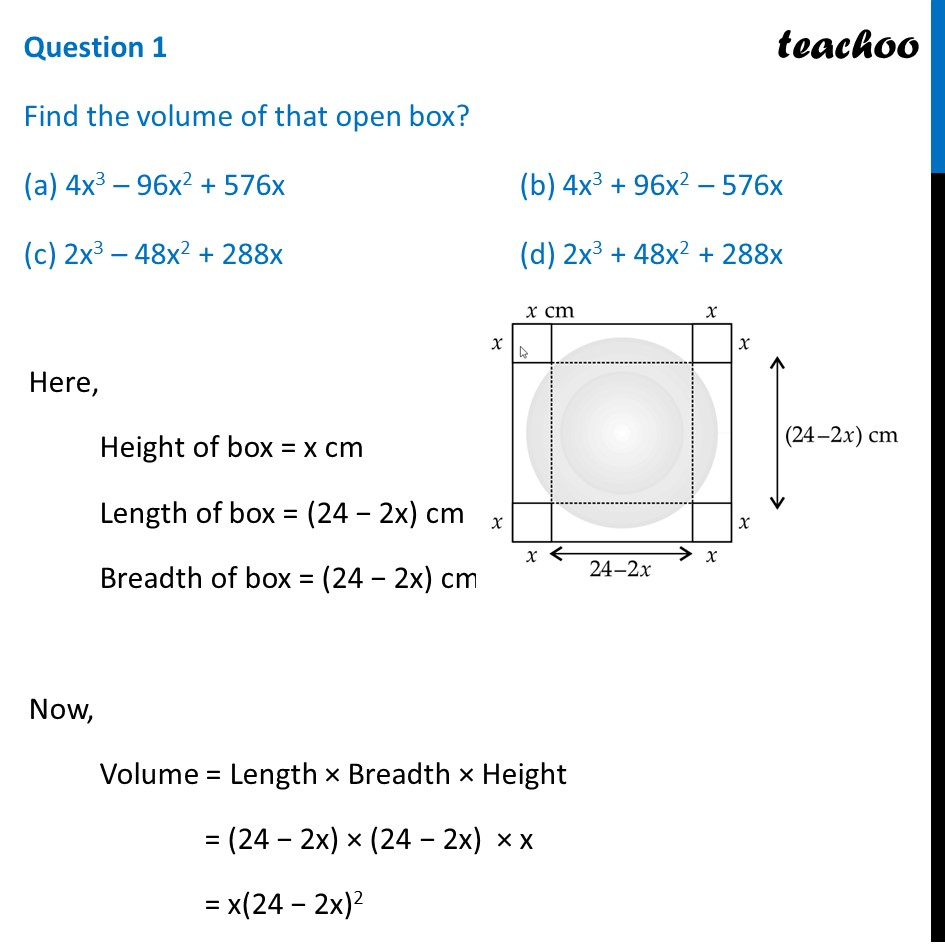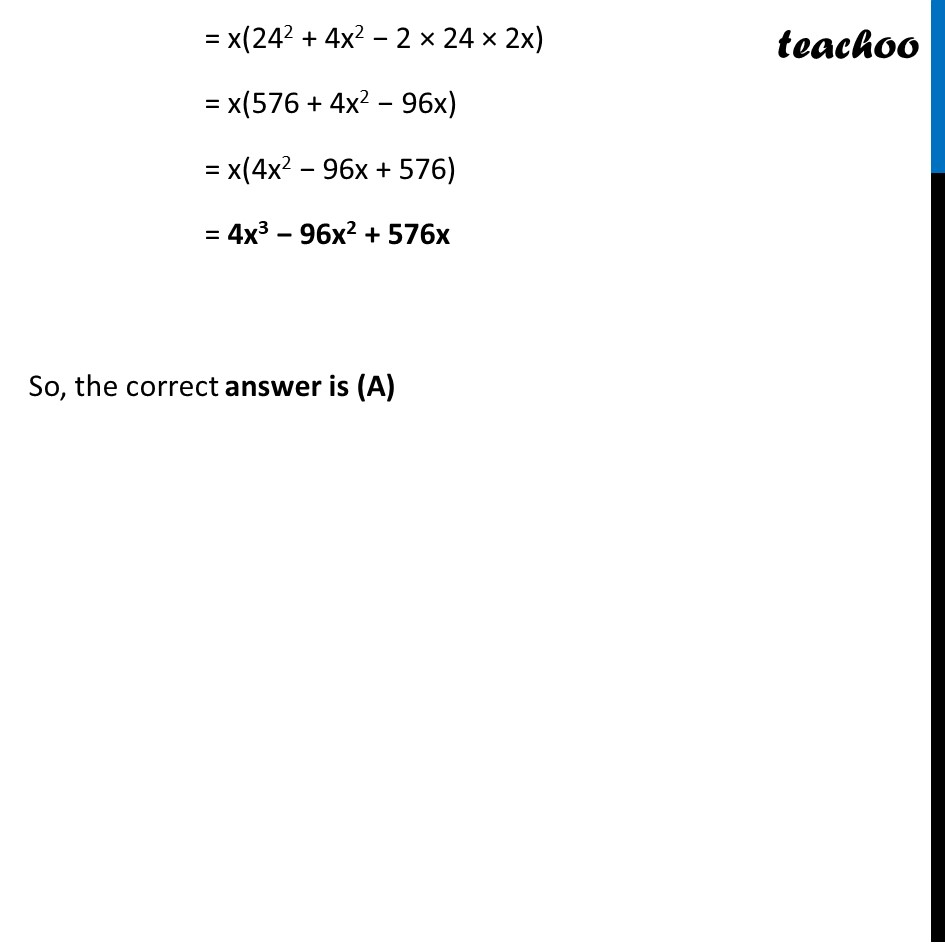## (d) 6(x 2 – 8x + 24)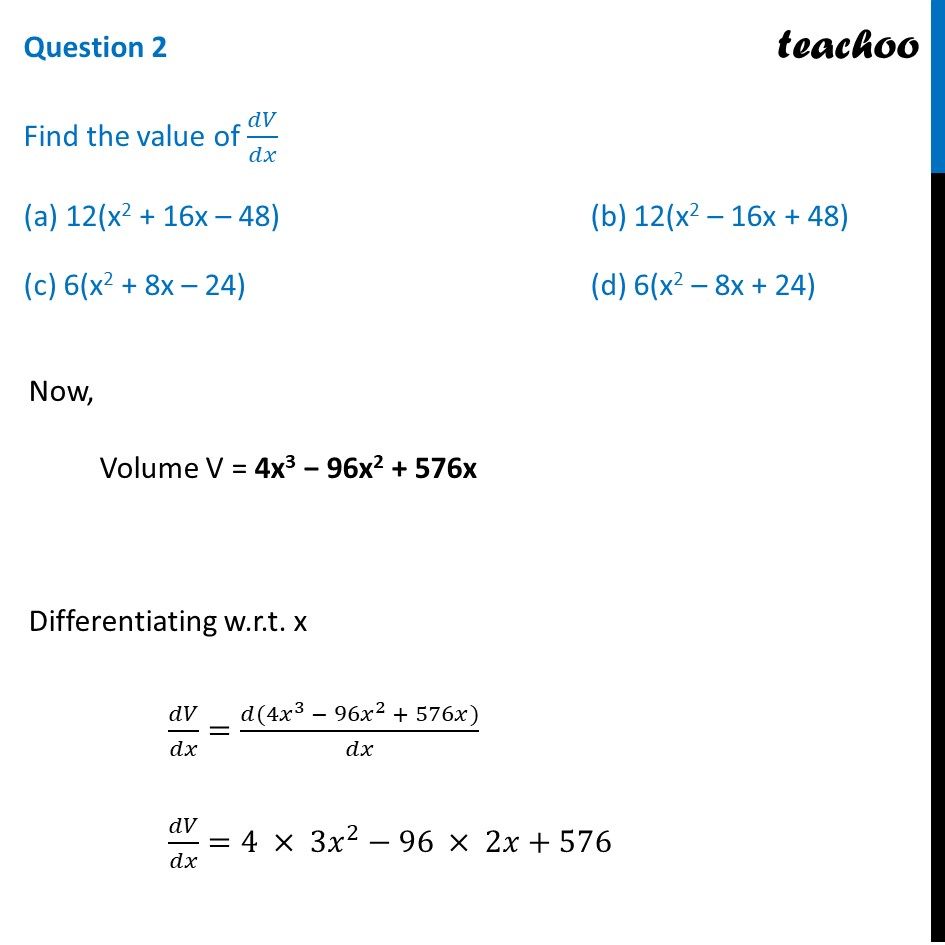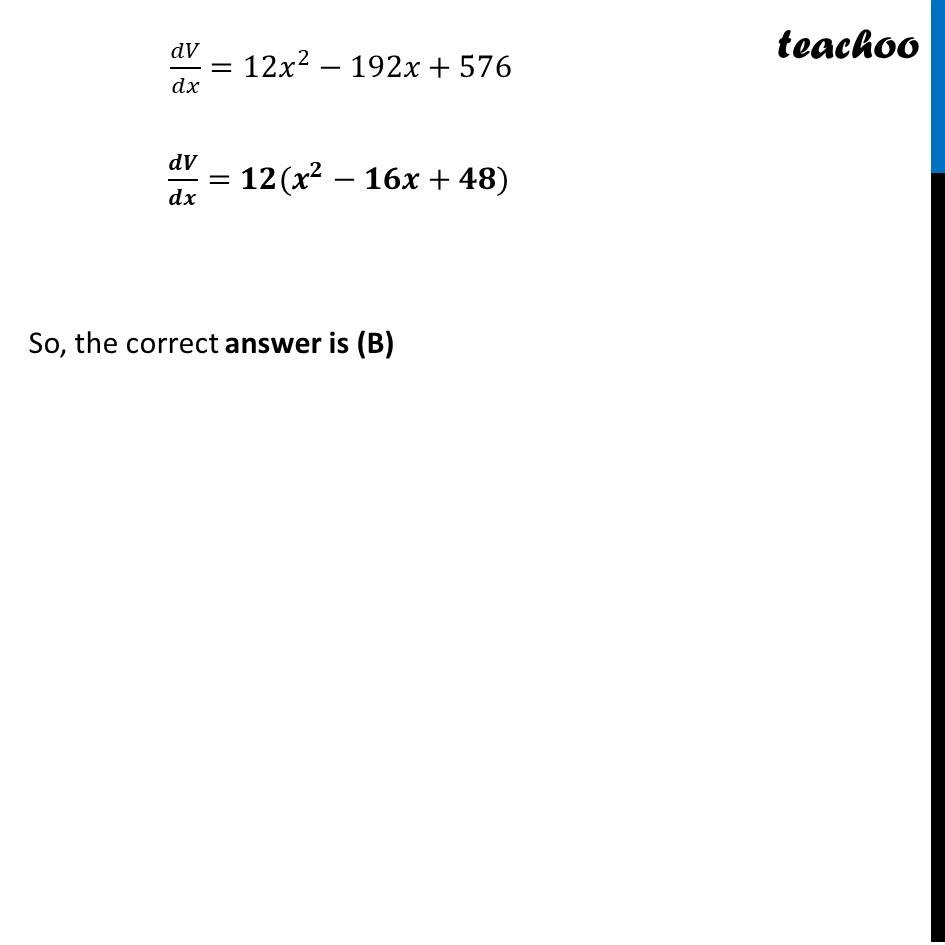## (d) 12(x + 4)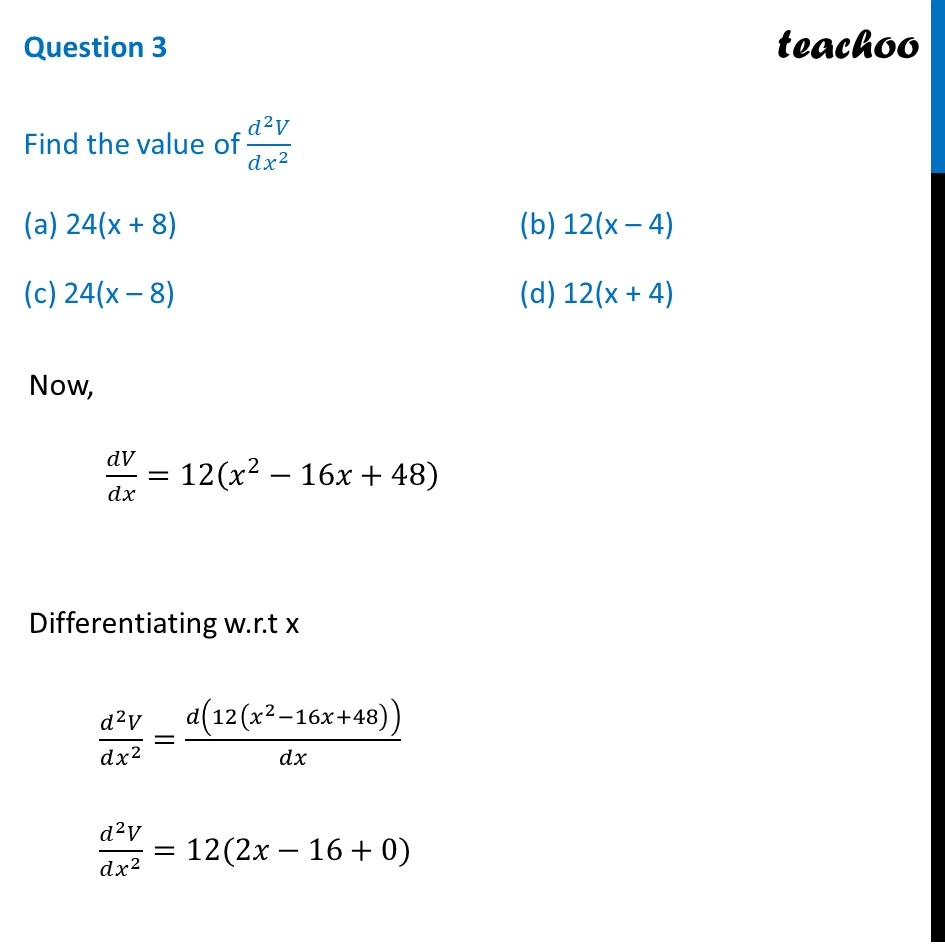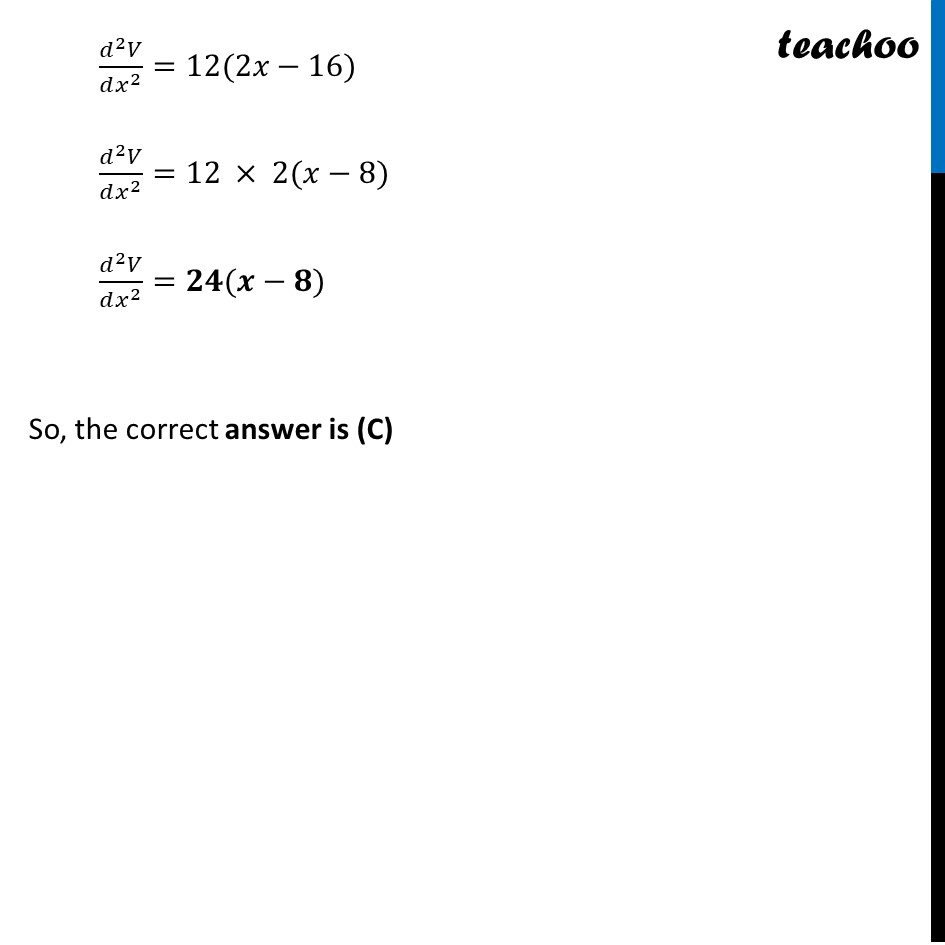## (d) 4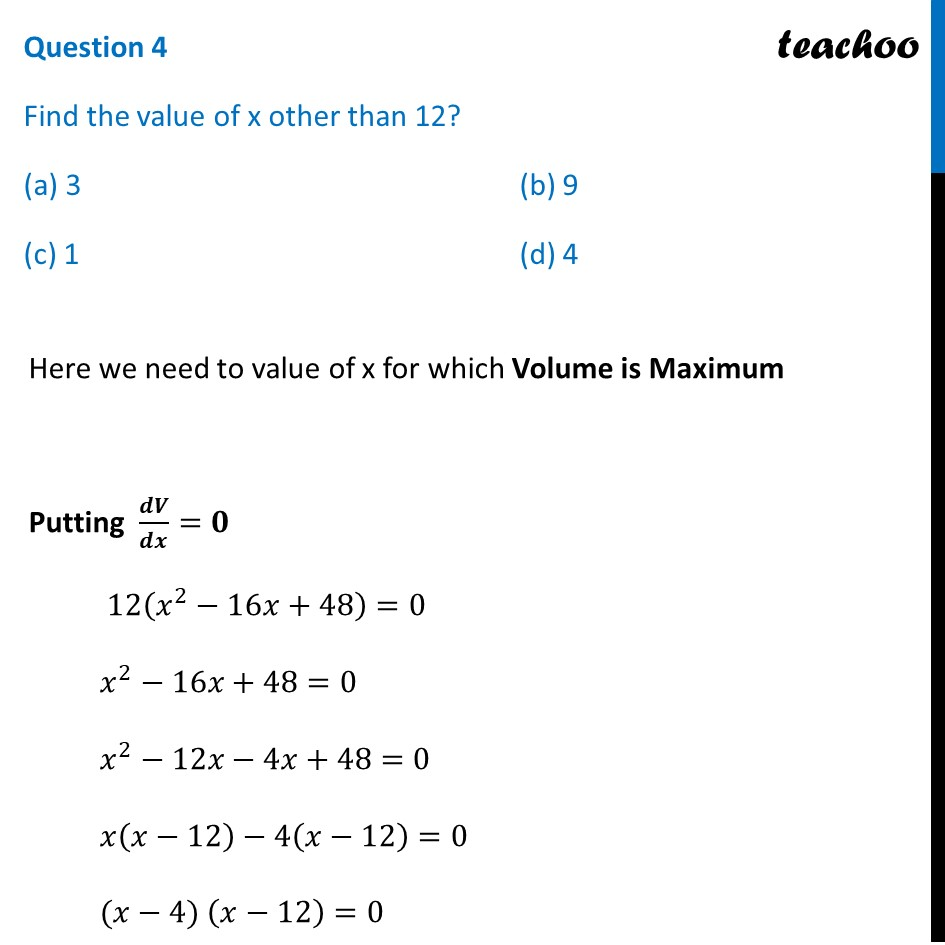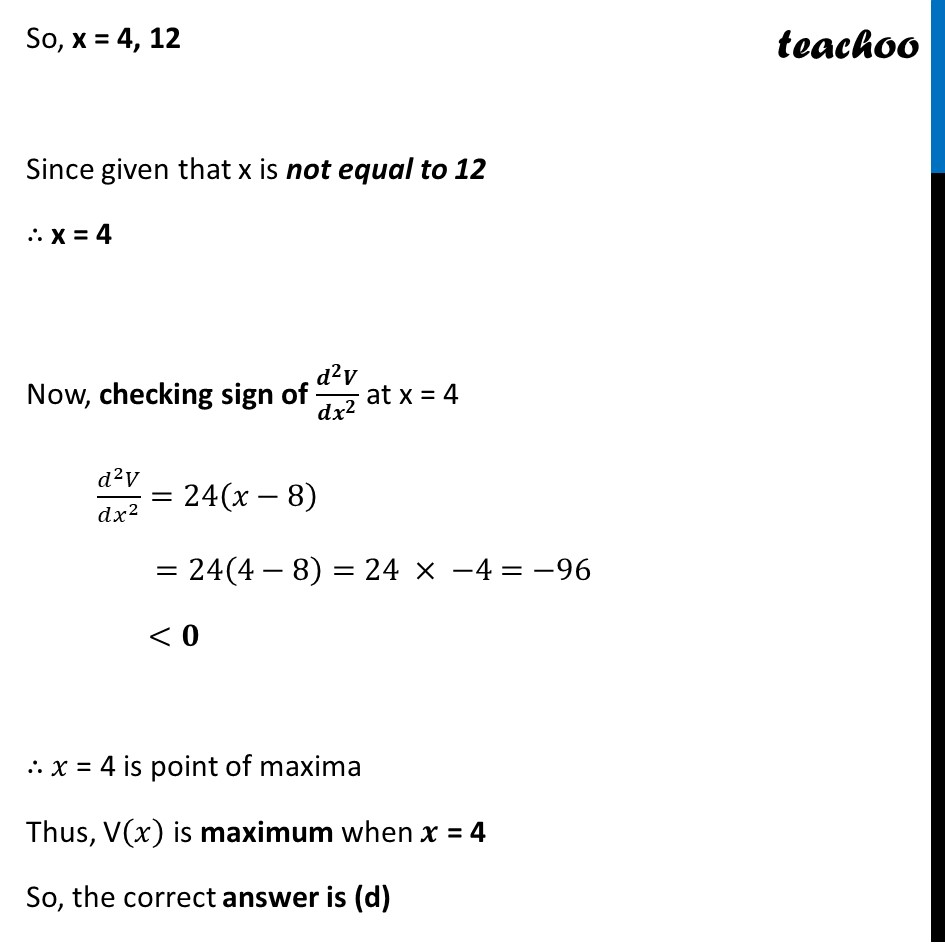## (d) 4 cm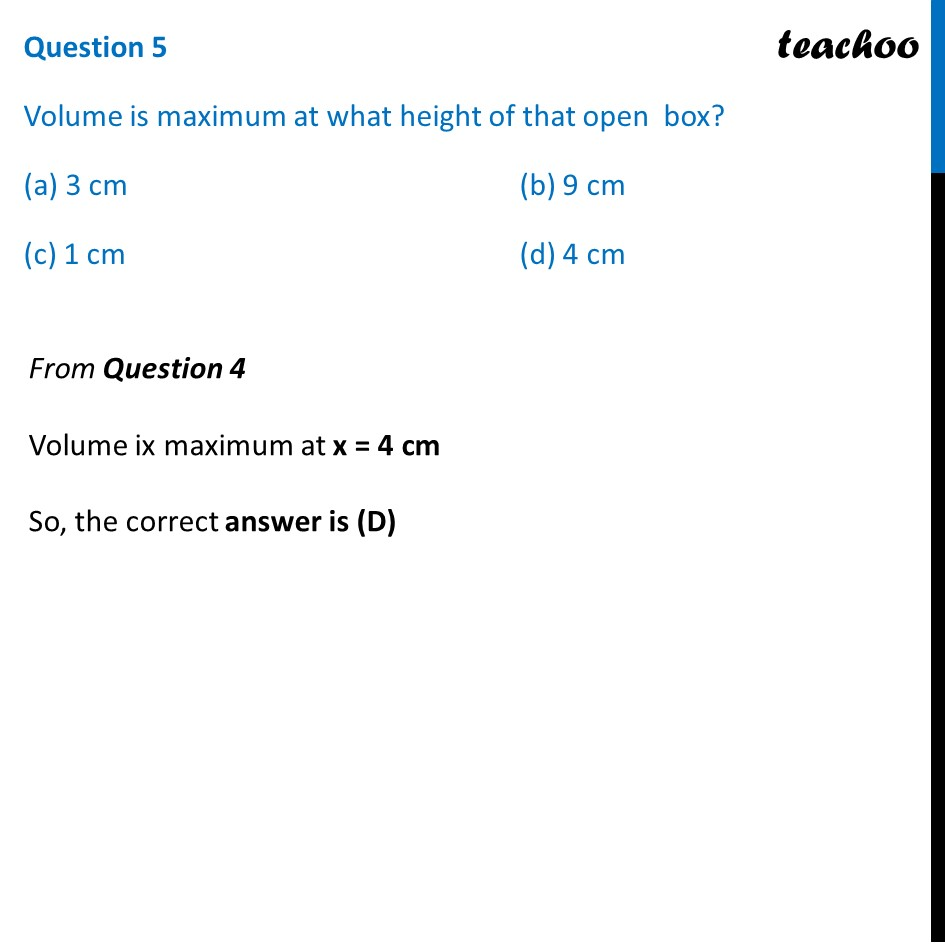1. Chapter 6 Class 12 Application of Derivatives (Term 1)
2. Serial order wise
3. Case Based Questions (MCQ)

Transcript

Question An open box is to be made out of a piece of cardboard measuring (24 cm × 24 cm) by cutting of equal squares from the corners and turning up the sides. Based on the above information answer the following questions: Question 1 Find the volume of that open box? (a) 4x3 – 96x2 + 576x (b) 4x3 + 96x2 – 576x (c) 2x3 – 48x2 + 288x (d) 2x3 + 48x2 + 288x Here, Height of box = x cm Length of box = (24 − 2x) cm Breadth of box = (24 − 2x) cm Now, Volume = Length × Breadth × Height = (24 − 2x) × (24 − 2x) × x = x(24 − 2x)2 = x(242 + 4x2 − 2 × 24 × 2x) = x(576 + 4x2 − 96x) = x(4x2 − 96x + 576) = 4x3 − 96x2 + 576x So, the correct answer is (A) Question 2 Find the value of 𝑑𝑉/𝑑𝑥 (a) 12(x2 + 16x – 48) (b) 12(x2 – 16x + 48) (c) 6(x2 + 8x – 24) (d) 6(x2 – 8x + 24) Now, Volume V = 4x3 − 96x2 + 576x Differentiating w.r.t. x 𝑑𝑉/𝑑𝑥=(𝑑(4𝑥^3 − 96𝑥^2 + 576𝑥))/𝑑𝑥 𝑑𝑉/𝑑𝑥=4 × 3𝑥^2−96 × 2𝑥+576 𝑑𝑉/𝑑𝑥=12𝑥^2−192𝑥+576 𝒅𝑽/𝒅𝒙=𝟏𝟐(𝒙^𝟐−𝟏𝟔𝒙+𝟒𝟖) So, the correct answer is (B) Question 3 Find the value of (𝑑^2 𝑉)/(𝑑𝑥^2 ) (a) 24(x + 8) (b) 12(x – 4) (c) 24(x – 8) (d) 12(x + 4) Now, 𝑑𝑉/𝑑𝑥=12(𝑥^2−16𝑥+48) Differentiating w.r.t x (𝑑^2 𝑉)/(𝑑𝑥^2 )=𝑑(12(𝑥^2−16𝑥+48))/𝑑𝑥 (𝑑^2 𝑉)/(𝑑𝑥^2 )=12(2𝑥−16+0) (𝑑^2 𝑉)/(𝑑𝑥^2 )=12(2𝑥−16) (𝑑^2 𝑉)/(𝑑𝑥^2 )=12 × 2(𝑥−8) (𝑑^2 𝑉)/(𝑑𝑥^2 )=𝟐𝟒(𝒙−𝟖) So, the correct answer is (C) Question 4 Find the value of x other than 12? (a) 3 (b) 9 (c) 1 (d) 4 Here we need to value of x for which Volume is Maximum Putting 𝒅𝑽/𝒅𝒙=𝟎 12(𝑥^2−16𝑥+48)=0 𝑥^2−16𝑥+48=0 𝑥^2−12𝑥−4𝑥+48=0 𝑥(𝑥−12)−4(𝑥−12)=0 (𝑥−4) (𝑥−12)=0 So, x = 4, 12 Now, checking sign of Volume V = 4x3 − 96x2 + 576x = 4x(x2 − 24x + 144) For x = 1 Volume = 4(1) (12 − 24(1) + 144) So, the correct answer is (D) Question 5 Volume is maximum at what height of that open box? (a) 3 cm (b) 9 cm (c) 1 cm (d) 4 cm From Question 4 Volume ix maximum at x = 4 cm So, the correct answer is (D)